# Texas Go Math Grade 3 Lesson 18.7 Answer Key Customary Units for Weight

Refer to our Texas Go Math Grade 3 Answer Key Pdf to score good marks in the exams. Test yourself by practicing the problems from Texas Go Math Grade 3 Lesson 18.7 Answer Key Customary Units for Weight.

## Texas Go Math Grade 3 Lesson 18.7 Answer Key Customary Units for Weight

Unlock the Problem

Weight is the measure of how heavy an object is. Customary units for weight include ounce (oz) and pound (lb).

Circle the correct word to complete the sentences.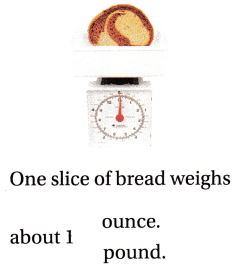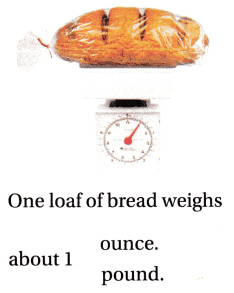Math Talk
Mathematical Processes

Suppose you weigh a bag of potatoes in pounds, and then in ounces. Which number would be greater, the number of ounces or the number of pounds? Explain.
Pounds are heavier than ounces.

Try This!

Sixteen ounces and one pound equal the same weight. Finish the drawing of slices of bread to show how ounces and pounds are related.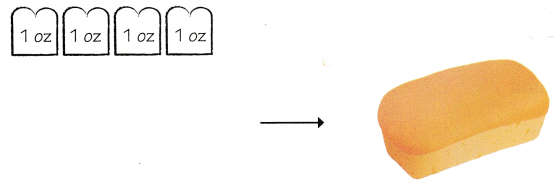________ ounces in ________ pound
16 ounces in 1 pound.

Explanation:Activity

Materials; scale, classroom objects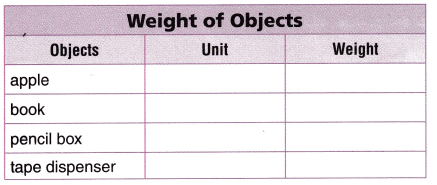Remember
Remember to include the units when recording the weights in the table.

STEP 1:
Use a scale to measure to the nearest

STEP 2:
Repeat for each object.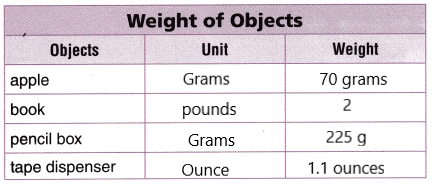Share and Show

Question 1.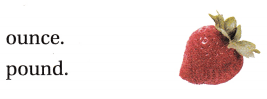Ounce

Explanation:Choose the unit you would use to measure the weight. Write ounce or pound.

Question 2.Ounce

Question 3.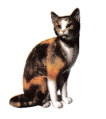Poundounce

Question 5.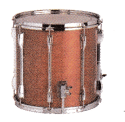Pound

Find an object in the classroom to match the description. Draw and label the object.

Question 6.
greater than 1 pound
A Shoe

Explanation:
A shoe is greater than 1 pound

Question 7.
less than 1 pound

Unlock the Problem

Question 8.
H.O.T. Multi-Step Jared has a canister that has 48 ounces of home-made trail mix. He wants to package the mix in bags that each hold 1 pound of mix. How many bags can Jared fill?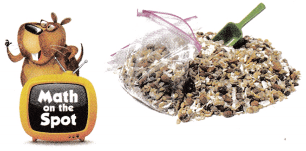a. What do you need to find?
I need to find the number of bags that 48 ounces of the mix would fill.

b. What are two ways to describe the amount the bags can hold?
1 pound or 16 ounces.

c. Representations Show the steps you used to solve the problem.
I drew 48 counters to show 48 ounces. Then I circled groups of 16 to show one pound.

Explanation: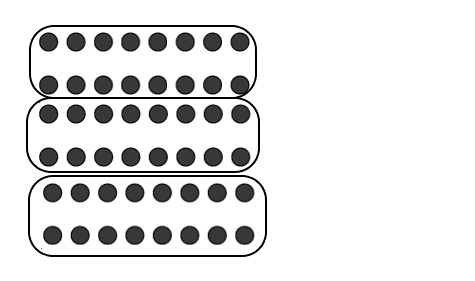d. Complete the sentences.
Sixteen ounces is the same weight as __________.
Each bag holds __________ ounces of mix.
48 ounces can be separated into __________ groups of __________ ounces each.
So, Jared can fill __________ bags with 1 pound of trail mix each.
Sixteen ounces is the same weight as 1 pound.
Each bag holds 16 ounces of mix.
48 ounces can be separated into 3 groups of 16 ounces each.
Jared can fill 3 bags with 1 pound of trail mix each.

Question 9.
H.O.T. Use Math Language Hank says that 32 ounces is the same as 3 pounds. Does this statement make sense? Explain.
No

Explanation:
Here 16 ounces equals 1 pound, 32 ounces equals 2 pounds, which is not equal to 3 pounds.

Fill in the bubble for the correct answer choice.

Question 10.
A watermelon weighs 6 pounds. Which item would be about the same weight as the watermelon?
(A) 10-ounce package of straws
(B) 8 slices of cheese pizza
(C) 6 erasers
(D) 480 quarters
480 quarters

Explanation:
The item 480 quarters would be about the same weight as the watermelon.

Question 11.
Brianna is building a doghouse. Each board weighs about 10 ounces. She lifts 8 boards at a time. About how much does Brianna lift?
(A) 3 ounces
(B) 4 ounces
(C) 5 pounds
(D) 2 pounds
5 pounds

Explanation:
1 pound = 16 ounces
Each board weighs 10 ounces
Brianna lifts 8 boards at a time.
Therefore Brianna lifts $$\frac{10×8}{16}$$. Brianna lifted 5 pounds.

Question 12.
Multi-Step Sandra and Richelle baked 2 cakes. They cut both cakes into 8 equal slices. Each slice weights about 2 ounces. Which is the combined weight of the cakes?
(A) 4 ounces
(B) 2 pounds
(C) 16 ounces
(D) 1 pound
2 pounds

Explanation:
The combined weight of the cake is 2 pounds.

Texas Test Prep

Question 13.
There are 4 baseball caps at a store. Each cap weighs 8 ounces. How much do the caps weigh in all?
(A) 8 ounces
(B) 10 ounces
(C) 2 pounds
(D) 4 pounds
2 pounds

Explanation:
The caps weigh 2 pounds weigh in all.

### Texas Go Math Grade 3 Lesson 18.7 Homework and Practice Answer Key

Choose the unit you would use to measure the weight. Write ounce or pound.

Question 1.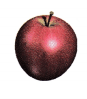Ounce

Question 2.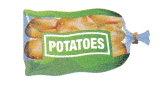Pound

Question 3.Ounce

Question 4.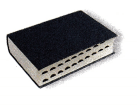Pound

Question 5.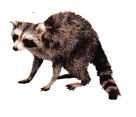Pound

Question 6.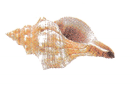ounce

Problem Solving

Question 7.
Multi-Step Janet buys 6 bags of potatoes. Each bag holds 8 ounces. How many pounds of potatoes does Janet buy?
3 pounds

Explanation:
Janet bought 3 pounds of potatoes.

Question 8.
Multi-Step Pedro buys 2 pounds 8 ounces of tomatoes. How many ounces of tomatoes does Pedro buy?
40 ounces

Explanation:
Pedro bought 40 ounces of tomatoes.

Question 9.
Sonny has a bag that holds 32 ounces of rice. He wants to divide the rice into boxes that hold 1 pound each. How many boxes can Jared fill? Draw a picture to solve the problem. Explain how you solved the problem.
2 boxes

Explanation:
I drew 32 counters to show 32 ounces. Then I circled groups of 16 to show one pound. So, 32 ounces equals 2 pounds.

Lesson Check

Question 10.
A small paperback book weighs about 10 ounces. Which item would be about the same weight as the paperback book?
(A) a slice of pizza
(B) a pound of potatoes
(C) a quart of milk
A slice of pizza

Explanation:
A slice of pizza would be about the same weight as the paperback book.

Go Math Answer Key Customary Unit of Weight Grade 3 Question 11.
A bag of onions weighs about 3 pounds. Which items would be about the same weight as the bag of onions?
(A) 20 balloons
(B) 5 turkeys
(C) 10 tomatoes
(D) 15 feathers
10 tomatoes

Explanation:
10 tomatoes would have about the same weight as the bag of onions.

Question 12.
Multi-Step A worker loads boxes of sugar. Each box weighs 16 ounces. The worker loads 20 boxes at one time. How many pounds of sugar does the worker load?
(A) 36 pounds
(B) 20 pounds
(C) 16 pounds
(D) 10 pounds
20 pounds

Explanation:
The worker load has 20 pounds of sugar.

Question 13.
Multi-Step Otis bakes 8 mini loaves of bread. Each loaf weighs about 8 ounces. About how many pounds of bread does Otis bake?
(A) 4 pounds
(B) 6 pounds
(C) 5 pounds
(D) 3 pounds
4 pounds

Explanation:
Otis bakes 4 pounds of bread.

Question 14.
Multi-Step Yogurt comes in containers that weigh 4 ounces. Each pack contains 6 yogurt containers. If Otis buys 2 packs of yogurt, how many pounds of yogurt does he buy?
(A) 4 pounds
(B) 2 pounds
(C) 3 pounds
(D) 5 pounds
3 pounds

Explanation:
He bought 3 pounds of yogurt.

Question 15.
Multi-Step There are 5 bananas in a bunch. Each banana weighs about 8 ounces. How many pounds and ounces does the bunch of bananas weigh?
(A) 2 pounds 8 ounces
(B) 1 pound 8 ounces
(C) 2 pounds 5 ounces
(D) 1 pound 5 ounces